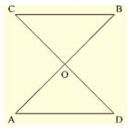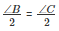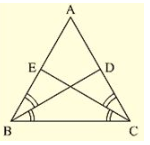Courses

# RD Sharma Solutions -Ex-10.2, Congruent Triangles, Class 9, Maths Class 9 Notes | EduRev

## Class 9 : RD Sharma Solutions -Ex-10.2, Congruent Triangles, Class 9, Maths Class 9 Notes | EduRev

The document RD Sharma Solutions -Ex-10.2, Congruent Triangles, Class 9, Maths Class 9 Notes | EduRev is a part of the Class 9 Course RD Sharma Solutions for Class 9 Mathematics.
All you need of Class 9 at this link: Class 9

Q. (1) In fig. (10).40, it is given that RT = TS, ∠ 1 = 2 ∠ 2 and 4 = 2 ∠ (3) Prove that ΔRBT ≅ ΔSAT.

Solution:

In the figure, given that

RT = TS                                                                          ……(i)

∠ 1 = 2 ∠ 2            ……(ii)

And ∠ 4 = 2 ∠ 3    ……(iii)

To prove that ΔRBT ≅ ΔSAT.

Let the point of intersection RB and SA be denoted by O

Since RB and SA intersect at O

∠ AOR = ∠ BOS          [Vertically opposite angles]

• ∠ 1 = ∠ 4
• 2 ∠ 2 = 2 ∠ 3 [From (ii) and (iii)]
• ∠ 2 = ∠ 3 ……(iv)

Now we have RT =TS in Δ TRS

Δ TRS is an isosceles triangle

∠ TRS = ∠ TSR              ……(v)

But we have

∠ TRS = ∠ TRB + ∠ 2                                                              ……(vi)

∠ TSR = ∠ TSA + ∠ 3                                                              ……(vii)

Putting (vi) and (vii) in (v) we get

∠ TRB + ∠ 2 =  ∠ TSA + ∠ B

=> ∠ TRB = ∠ TSA        [From (iv)]

Now consider Δ RBT and Δ SAT

RT = ST [From (i)]

∠ TRB = ∠ TSA            [From (iv)]  ∠ RTB = ∠ STA    [Common angle]

From ASA criterion of congruence, we have

Δ RBT = Δ SAT

Q. (2) Two lines AB and CD intersect at O such that BC is equal and parallel to AD. Prove that the lines AB and CD bisect at O.

Solution: Given that lines AB and CD Intersect at OWe have to prove that AB and CD bisect at O.

To prove this first we have to prove that Δ AOD ≅ Δ BOC

Q. (3) BD and CE are bisectors of ∠ B and ∠ C of an isosceles Δ ABC with AB = AC. Prove that BD = CE

Solution:

Given that Δ ABC is isosceles with AB = AC and BD and CE are bisectors of ∠ B and ∠ C We have to prove BD = CE

Since AB = AC

=> Δ ABC = Δ ACB ……(i)

[Angles opposite to equal sides are equal]

Since BD and CE are bisectors of ∠ B and ∠ C

• ∠ ABD = ∠ DBC = ∠ BCE = ECA =Now,

Consider Δ EBC = Δ DCB∠ EBC = ∠ DCB [∠ B = ∠ C] [From (i)]

BC = BC [Common side]

∠ BCE = ∠ CBD [From (ii)]

So, by ASA congruence criterion, we have Δ EBC ≅ Δ DCB

Now,

CE = BD     [Corresponding parts of congruent triangles we equal]

or, BD = CE

Hence proved

Since AD ∥ BC and transversal AB cuts at A and B respectively

∠ DAO = ∠ OBC  …….(ii) [alternate angle]

And similarly AD ∥ BC and transversal DC cuts at D and C respectively

∠ ADO = ∠ OBC                 ..…(iii) [alternate angle]

Since AB end CD intersect at O.

∠ AOD = ∠ BOC               [Vertically opposite angles]

Now consider Δ AOD and Δ BOD

∠ DAO = ∠ OBC [From (ii)]

And ∠ ADO = ∠ OCB  [From (iii)]

So, by ASA congruence criterion, we have

ΔAOD≅ΔBOC

Now,

AO= OB and DO = OC [Corresponding parts of congruent triangles are equal)

• Lines AB and CD bisect at O.

Hence proved

Offer running on EduRev: Apply code STAYHOME200 to get INR 200 off on our premium plan EduRev Infinity!

91 docs

,

,

,

,

,

,

,

,

,

,

,

,

,

,

,

,

,

,

,

,

,

,

,

,

,

,

,

,

,

,

;